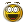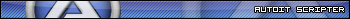Recommended Posts

Hi,

I`m trying to generate "fake passwords" that will be input inside notepad.

But I am having difficulty doing the following :

The first character must be A to Z, Uppercase.

The next 6 are numbers.

The last one is a to z Lowercase.

Any idea how to proceed?

Thank you

Edited by PinetSebastien

Share on other sites

Look ramdom function.

```for \$i=1 to 100
ConsoleWrite(RandomKey() & @CRLF)
Next

Func RandomKey()

For \$i=1 to 6
Next
EndFunc ```

Saludos

Edited by DanyfirexDanysys.comAutoIt...

Share on other sites

not clear what should be the last character

from A to z either upper or lowercase? or only lowercase?

EDIT:

if should be A to z , either upper or lowercase then use this in Danyfirex listing to get last character:

`\$cadena &= Chr(Random(65, 90, 1) + (32 * Random(0, 1, 1)))`
Edited by Chimp

small minds discuss people average minds discuss events great minds discuss ideas.... and use AutoIt....

Share on other sites

I did it in Excel with a few formulas and the RANDBETWEEN function. Seeing how you want to use notepad to store your values, it appears to me using Excel would be easier to go with.

Cell A1 has this formula: =C1&D1&E1&F1&G1&H1&I1&K1 (displays the password string you want)

Cell B1 has this formula: =RANDBETWEEN(1,26)

Cell C1 has =IF(B1=1, "A",  IF(B1=2, "B",.....and so on to  IF(B1=26, "Z",""))))))...) (this gives the capital letter in the string)

Cell D1 thru I1 have the same formula: =RANDBETWEEN(0,9)

Cell J1 has this formula: =RANDBETWEEN(1,26)

Cell K1 has this formula: IF(J1=1, "a",IF(J1=2, "b",,.....and so on to  IF(J1=26, "z",""))))))...) (this gives the lower case letter in the string)

Just copy and paste the cells to other rows to generate as many as you want. Just copy and past the values generated by column A to a different row to make the values static. When you need more passwords, just press F9

Edited by MBALZESHARI